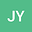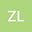Kirchhoff index of a linear hexagonal chain
••• Juan Yan,
• zhenzhen Lou
Juan Yan
Lishui University

Corresponding Author:[email protected]

Author Profilezhenzhen Lou
University of Shanghai for Science and Technology
Author Profile## Abstract

Let $H_n$ be a linear hexagonal chain with $n$ hexagons. In this paper, we give a decomposition theorem of Laplacian polynomial of weighted graphs and obtain that the Laplacian spectrum of $H_n$ consists of the eigenvalues of a symmetric tridiagonal matrices of order $4n+2$ and the Laplacian eigenvalues of $2n$ $K_2s$. Together with the relationship between the roots and coefficients of the characteristic polynomials of the above matrices, explicit formula of the Kirchhoff index of $H_n$ is derived. We also give the number of spanning trees of $H_n$, and show that the Kirchhoff index of $H_n$ is approximately one half of its Wiener index.